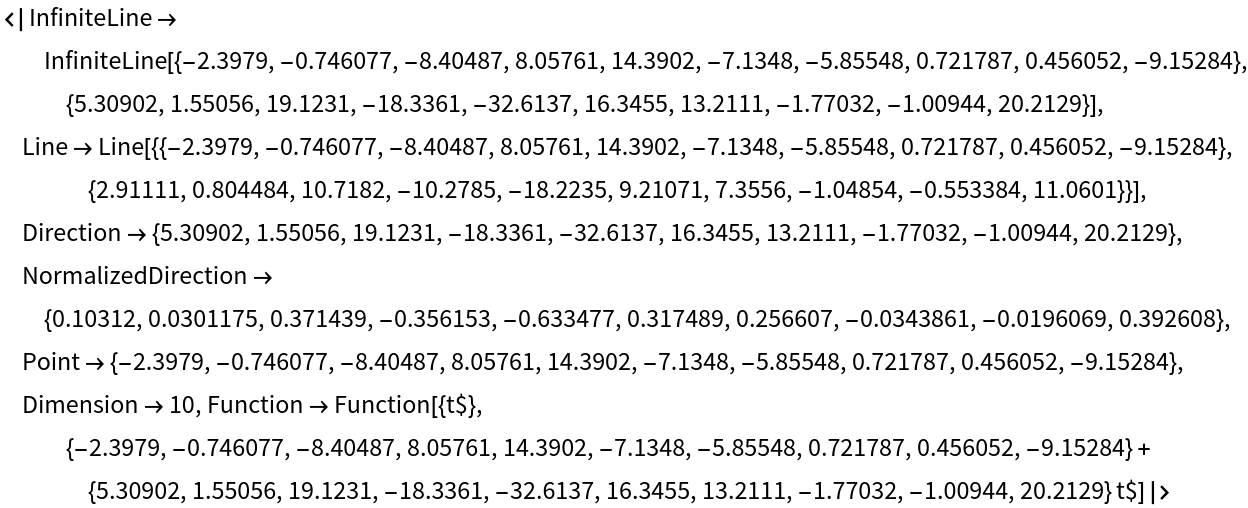Function Repository Resource:

LineFit

Fit a line to data points in n-dimensional space

Contributed by: Sander Huisman
 ResourceFunction["LineFit"][pts] returns a Line that fits best through the points pts. ResourceFunction["LineFit"][pts,"Association"] returns an Association containing properties of the best fit line.

Details

The returned function in the Association will correspond to a domain of 0 to 1.
The best fit is determined by minimizing the sum of squares of distances from the points to the best-fit line.

Examples

Basic Examples (2)

Fit a line in 2D:

 In:=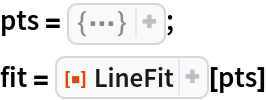Out=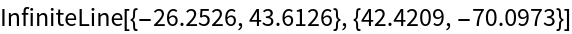Compare the points and the fit:

 In:=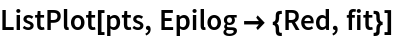Out=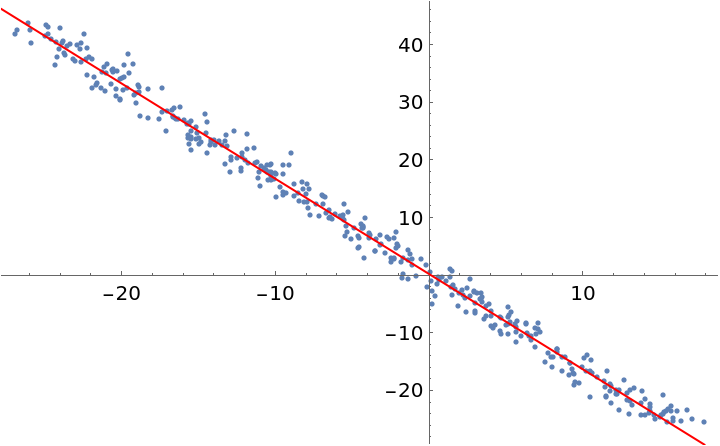Scope (2)

Do a fit with points in 3D:

 In:=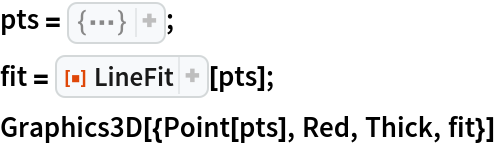Out=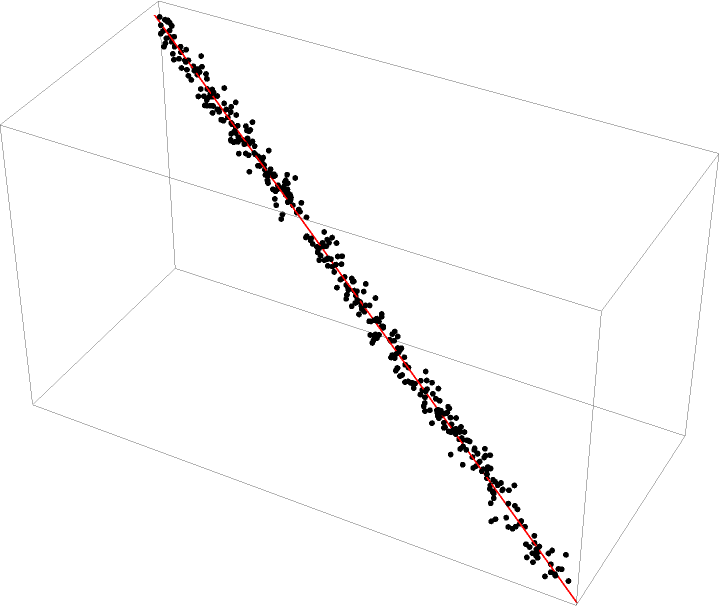Get a Dataset with all the fitting details:

 In:=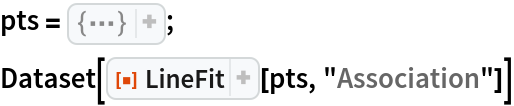Out=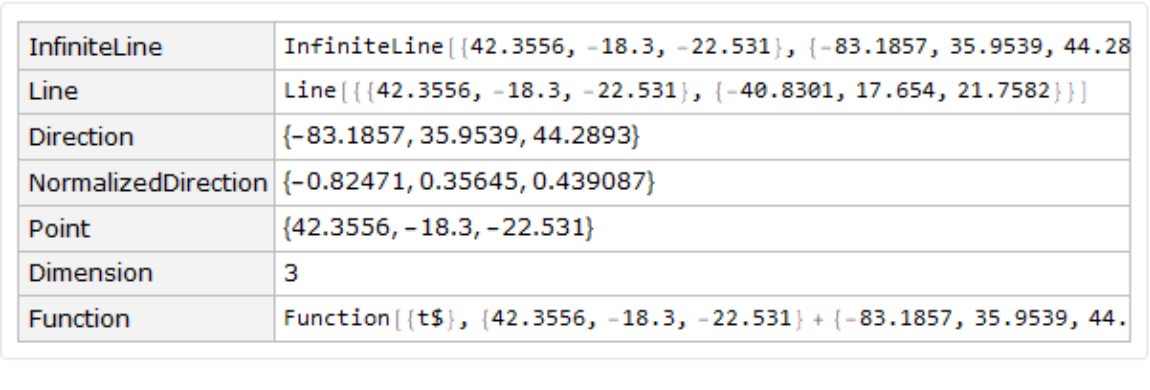Properties and Relations (2)

Compare the result of LineFit with the result of computing the orthogonal fit through the definition:

 In:=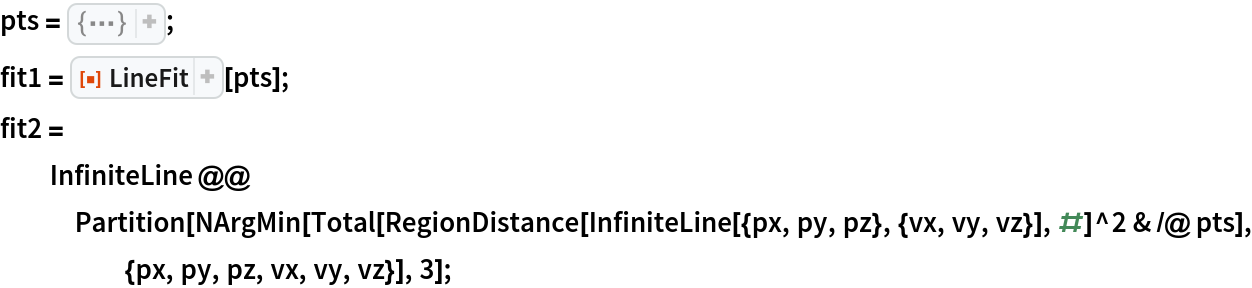In:=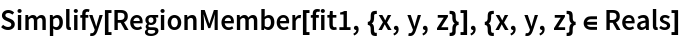Out=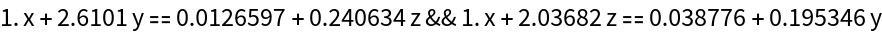In:=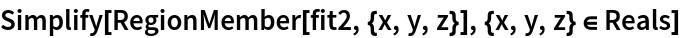Out=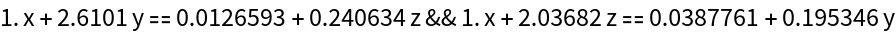The one-argument case of LineFit is equivalent to the resource function OrthogonalLineFit:

 In:=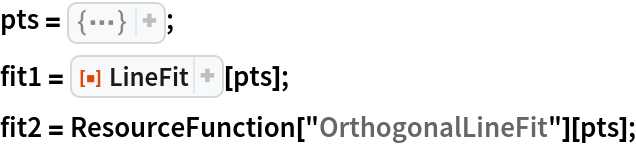In:=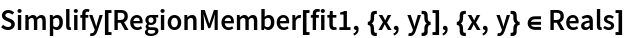Out=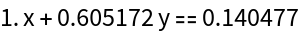In:=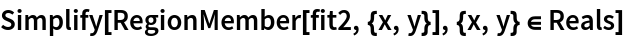Out=Possible Issues (3)

All points have to be numeric:

 In:=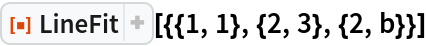Out=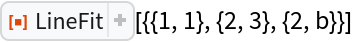All points have to be in the same dimension:

 In:=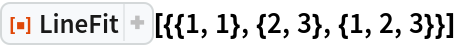Out=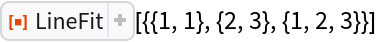Real numbers have to be used:

 In:=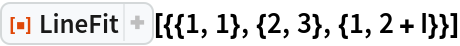Out=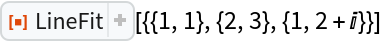Neat Examples (1)

Do a fit in 10D:

 In:=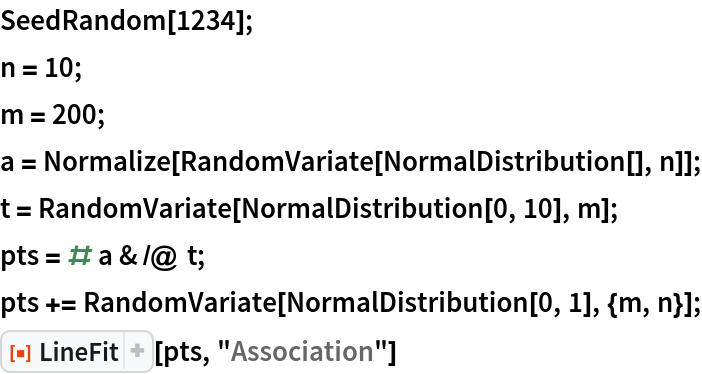Out=